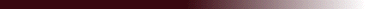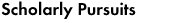## "A Hamiltonian Formulation for the Diffusion Equation"

### A Hamiltonian formulation is presented for the diffusion equation. Going beyond the conservation of property in diffusive transport, conservation is shown to apply in the sense of classical Hamiltonian dynamics, provided the equation is transformed with Hermitian wavelets. The characteristic equations, obtained previously for the wavelet-transformed diffusion equation, are the canonical equations corresponding to a time-independent Hamiltonian. The configuration variables are defined by the canonical structure and its invariants, while the momenta determine the evolution of the system. Irreversibility results from the finite-time escape of trajectories, initiating from the smallest scales and eroding increasingly larger scales. However, this scale-dependent erosion of initial conditions does not necessarily imply memory loss.

Physical Review E 55,1590-1599 (1997).

Jacques Lewalle, jlewalle@syr.edu